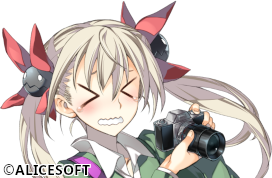# The PMS image format (8-bit)

PMS8 is an 8-bit indexed image format with compression. There is also a 16-bit direct color variant (PMS16). Both formats begin with the same header structure:

``````struct pms_header {
BYTE magic;    // "PM"
LE16 version;     // PMS version
BYTE bpp;         // bits per pixel, 8 or 16
BYTE alpha_bpp;   // alpha channel bit-depth, if exists
BYTE sf;          // sprite flag (not used?)
LE16 bf;          // palette bank
LE32 x;           // display location x
LE32 y;           // display location y
LE32 width;       // image width
LE32 height;      // image height
LE32 data_off;    // offset to image data
LE32 palette_off; // offset to palette or alpha
LE32 comment_off; // offset to comment
};``````

For PMS8, `bpp` is 8 and there is no alpha channel. The image data can be found at `data_off`, and the palette at `palette_off`. The palette is composed of 256 3-byte RGB values:

``````struct rgb {
BYTE red;
BYTE green;
BYTE blue;
} palette;``````

The image data is compressed using a few techniques. Refer to the commented source code below (adapted from xsystem35) to see how it works.

``````struct pms_cg {
struct {
uint8_t r;
uint8_t g;
uint8_t b;
} pal;
uint8_t *image;
int width;
int height;
}

/* Convert PMS8 image to 8-bit indexed bitmap. */
static int pms8_extract(uint8_t *pic, struct rgb *pal, uint8_t *data, size_t size)
{
// check for PMS8 format
if (data != 'P' || data != 'M' || data != 8 || size < 40)
return NULL;

const int width       = LittleEndian_getDW(data, 24);
const int height      = LittleEndian_getDW(data, 28);
const int image_off   = LittleEndian_getDW(data, 32);
const int palette_off = LittleEndian_getDW(data, 36);

// check dimensions/offsets
if (width < 0 || height < 0)
return NULL;
if (palette_off + 256*3 >= size || image_off >= size)
return NULL;

// allocate memory for bitmap
struct pms_cg *cg = calloc(1, sizeof(struct pms_cg));
cg->image = malloc((width + 10) * (height + 10)); // +10: margin for broken CGs
cg->width = width;
cg->height = height;

uint8_t p = data + palette_off;
for (int i = 0; i < 256; i++) {
cg->pal[i].r = *p++;
cg->pal[i].g = *p++;
cg->pal[i].b = *p++;
}

int n, c0, c1;
uint8_t *b = data + image_off;
uint8_t *pic = cg->image;

// for each line...
for (int y = 0; y < height; y ++) {
// for each pixel...
for (int x = 0; x < width; ) {
// check for corrupt CG
// NOTE: this is not robust, since we could read up to 4 bytes below
if (b - data >= size) {
free(cg->image);
free(cg);
return NULL;
}
int loc = y * width + x;
c0 = *b++;
// non-command byte: read 1 pixel into buffer
if (c0 <= 0xf7) {
pic[loc] = c0;
x++;
}
// copy n+3 pixels from previous line
else if (c0 == 0xff) {
n = (*b++) + 3;
x += n;
memcpy(pic + loc, pic + loc - width, n);
}
// copy n+3 pixels from 2 lines previous
else if (c0 == 0xfe) {
n = (*b++) + 3;
x += n;
memcpy(pic + loc, pic + loc - width * 2, n);
}
// repeat 1 pixel n+4 times (1-byte RLE)
else if (c0 == 0xfd) {
n = (*b++) + 4;
x += n;
c0 = *b++;
memset(pic + loc, c0, n);
}
// repeast a sequence of 2 pixels n+3 times (2-byte RLE)
else if (c0 == 0xfc) {
n = ((*b++) + 3)  * 2;
x += n;
c0 = *b++;
c1 = *b++;
for (int i = 0; i < n; i+=2) {
pic[loc+i]   = c0;
pic[loc+i+1] = c1;
}
}
// not sure why this exists, probably padding
else {
pic[loc] = *b++;
x++;
}
}
}
return cg;
}``````